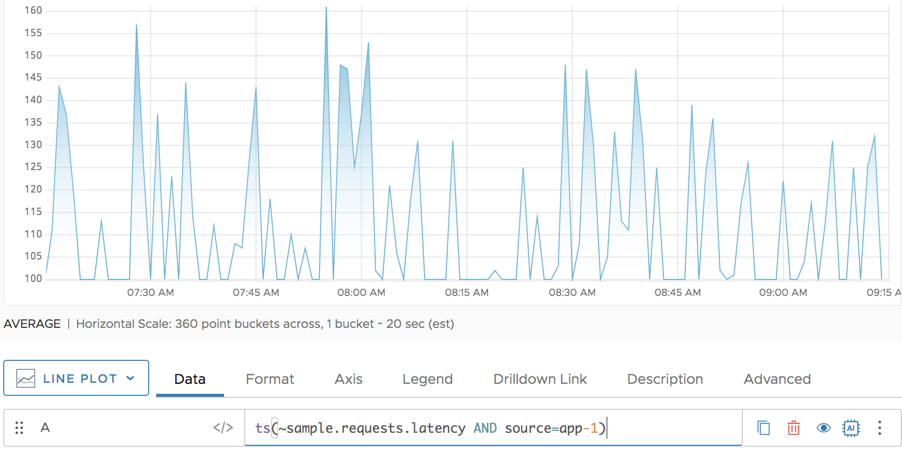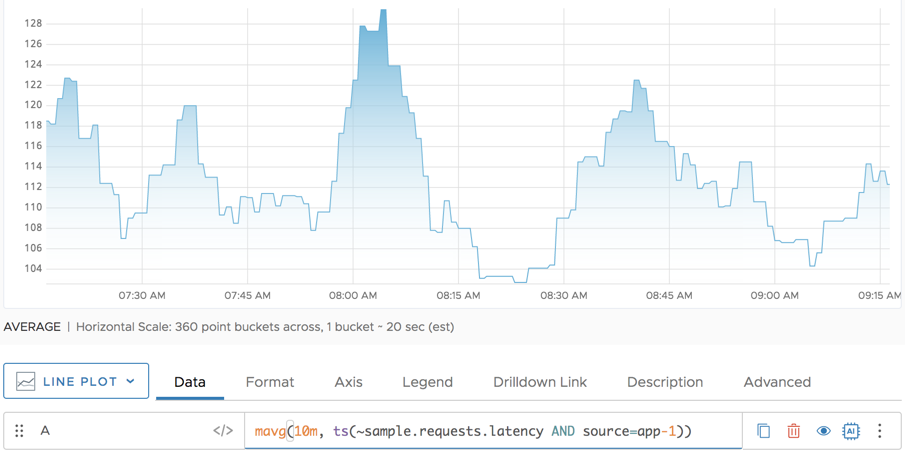Reference to the mavg() function

## Summary

``````mavg(<timeWindow>, <tsExpression>)
``````

Returns the moving average of each time series over the specified time window.

## Parameters

ParameterDescription
timeWindow Amount of time in the moving time window. You can specify a time measurement based on the clock or calendar (1s, 1m, 1h, 1d, 1w), the window length (1vw) of the chart, or the bucket size (1bw) of the chart. Default is minutes if the unit is not specified.
tsExpression Expression that describes the time series you want moving averages for.

## Description

The `mavg()` function computes the moving average of each time series over a shifting time window. For example, `mavg(60m, ts(my.metric))` returns, at each point, the average of the data values over the previous 60 minutes for each specified time series.

Here’s how to select your averaging function:

• Use `mavg()` to see the moving average in a specified time window.
• Use `avg()` to see the average (the mean).
• Use `mmedian()` to see the median. Using `mmedian()` is preferred if there are a lot of outliers.
• Use `mpercentile()` with a percentile of 50 to see the moving median.

## Example

Let’s say you have the following expression:

`mavg(48h, ts(my.metric))`

Assume `ts(my.metric)` reports data values once per hour, but only started reporting data 24 hours ago. `mavg()` will add up all reported points within the last 48 hours and divide by the number of reported points (not number of hours). So in that example `mavg()` divides by 24 not by 48, because technically, we have only 24 reported points within the last 48 hours.

The following example shows the requests latency for a single app server (app-1):And here’s what we see when we apply `mavg()`.## Caveats

At times, using `msum()` can get you the information you want. In that case, use `msum()` because performance is better.